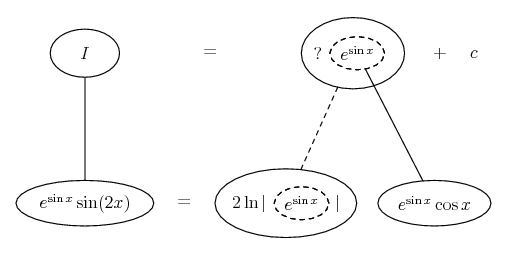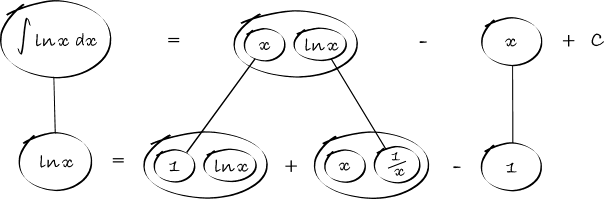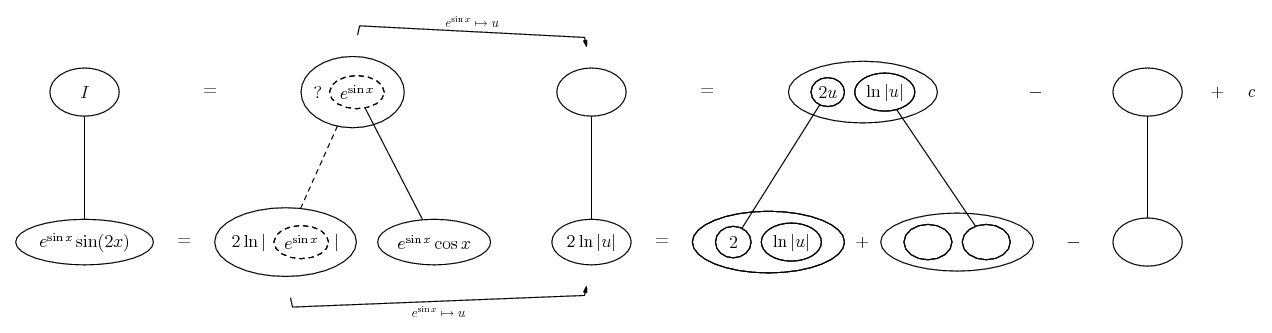1. ## Integral

Hi

I am having problems with the integral in the attached file.

I am guessing that I'm supposed to make some kind of variable substitution. I just can't figure out which and how.

Thankful for help.

Einar

2. $\displaystyle e^{\sin x} \sin(2x) = e^{\sin x} (2 \sin x \cos x)$

Try substituting with $\displaystyle u = e^{\sin x}$

Will post a little pic...

Just in case a picture helps...... where... is the chain rule. Straight continuous lines differentiate downwards (integrate up) with respect to x, and the straight dashed line similarly but with respect to the dashed balloon expression (the inner function of the composite which is subject to the chain rule).

To integrate $\displaystyle 2 \ln u$ i.e. 2 log of the dashed balloon i.e. to travel up the straight dashed line, use parts. The basic pattern for integrating ln is... where... the product rule. Straight continuous lines again differentiating downwards (integrating up) with respect to the main variable. So, putting it all together... (may be a couple of minutes, try and beat me to it)

Spoiler:... maybe not the very most simplifying example of balloontegrating - but I find it helps convey the logic of the normal method (which would involve switching dx -> du... someone may want to do that). A few blanks left to fill in - try to finish off the vaguely clockwise journey?
_________________________________________

Don't integrate - balloontegrate!

Balloon Calculus; standard integrals, derivatives and methods

Balloon Calculus Drawing with LaTeX and Asymptote!

3. Thanks a lot, i think iv'e got it now =)

#### Search Tags

integral